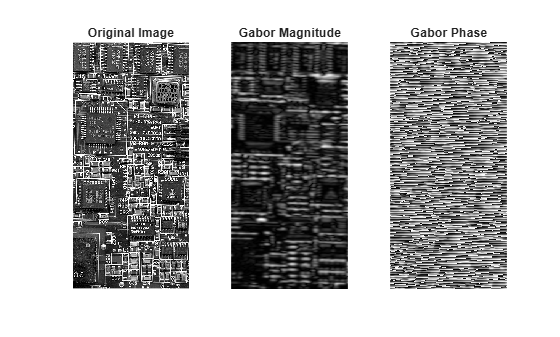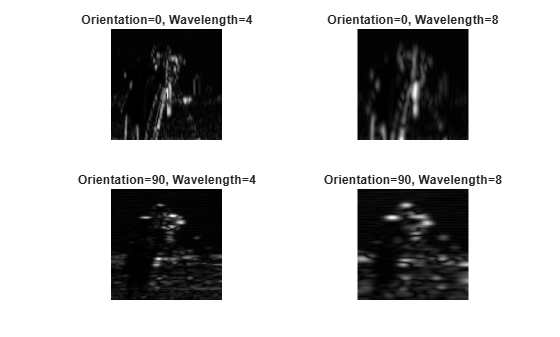# imgaborfilt

Apply Gabor filter or set of filters to 2-D image

## Syntax

``````[mag,phase] = imgaborfilt(A,wavelength,orientation)``````
``[mag,phase] = imgaborfilt(A,wavelength,orientation,Name,Value)``
``````[mag,phase] = imgaborfilt(A,gaborbank)``````

## Description

example

``````[mag,phase] = imgaborfilt(A,wavelength,orientation)``` computes the magnitude and phase response of a Gabor filter for the input grayscale image `A`. `wavelength` describes the wavelength in pixels/cycle of the sinusoidal carrier. `orientation` is the orientation of the filter in degrees. The output `mag` and `phase` are the magnitude and phase responses of the Gabor filter.```
````[mag,phase] = imgaborfilt(A,wavelength,orientation,Name,Value)` applies a single Gabor filter using name-value pairs to control various aspects of filtering.```

example

``````[mag,phase] = imgaborfilt(A,gaborbank)``` applies the array of Gabor filters, `gaborbank`, to the input image `A`. `gaborbank` is a 1-by-p array of Gabor objects, called a filter bank. `mag` and `phase` are image stacks where each plane in the stack corresponds to one of the outputs of the filter bank. For inputs of size `A`, the outputs `mag` and `phase` contain the magnitude and phase response for each filter in `gaborbank` and are of size m-by-n-by-p. Each plane in the magnitude and phase responses, `mag(:,:,ind),phase(:,:,ind)`, is the result of applying the Gabor filter of the same index, `gaborBank(ind)`.```

## Examples

collapse all

`I = imread('board.tif');`

Convert image to grayscale.

`I = rgb2gray(I);`

Apply Gabor filter to image.

```wavelength = 4; orientation = 90; [mag,phase] = imgaborfilt(I,wavelength,orientation);```

Display original image with plots of the magnitude and phase calculated by the Gabor filter.

```figure subplot(1,3,1); imshow(I); title('Original Image'); subplot(1,3,2); imshow(mag,[]) title('Gabor magnitude'); subplot(1,3,3); imshow(phase,[]); title('Gabor phase');````I = imread('cameraman.tif');`

Create array of Gabor filters, called a filter bank. This filter bank contains two orientations and two wavelengths.

`gaborArray = gabor([4 8],[0 90]);`

Apply filters to input image.

`gaborMag = imgaborfilt(I,gaborArray);`

Display results. The figure shows the magnitude response for each filter.

```figure subplot(2,2,1); for p = 1:4 subplot(2,2,p) imshow(gaborMag(:,:,p),[]); theta = gaborArray(p).Orientation; lambda = gaborArray(p).Wavelength; title(sprintf('Orientation=%d, Wavelength=%d',theta,lambda)); end```## Input Arguments

collapse all

2-D grayscale image, specified as a numeric matrix.

If the image contains `Inf`s or `NaN`s, the behavior of `imgaborfilt` is undefined because Gabor filtering is performed in the frequency domain.

For all input data types other than `single`, `imgaborfilt` performs the computation in `double`. Input images of type `single` are filtered in type `single`. Performance optimizations may result from casting the input image to `single` prior to calling `imgaborfilt`.

Data Types: `single` | `double` | `int8` | `int16` | `int32` | `int64` | `uint8` | `uint16` | `uint32` | `uint64`

Wavelength of the sinusoidal carrier, specified as a numeric scalar in the range `[2,Inf)`, in pixels/cycle.

Data Types: `single` | `double` | `int8` | `int16` | `int32` | `int64` | `uint8` | `uint16` | `uint32` | `uint64`

Orientation of filter in degrees, specified as a numeric scalar in the range `[0 360]`, where the orientation is defined as the normal direction to the sinusoidal plane wave.

Data Types: `single` | `double` | `int8` | `int16` | `int32` | `int64` | `uint8` | `uint16` | `uint32` | `uint64`

Array of Gabor filters, specified as a `gabor` object. You must use the `gabor` function to create an array of Gabor filters.

### Name-Value Pair Arguments

Specify optional comma-separated pairs of `Name,Value` arguments. `Name` is the argument name and `Value` is the corresponding value. `Name` must appear inside quotes. You can specify several name and value pair arguments in any order as `Name1,Value1,...,NameN,ValueN`.

Example: `[mag,phase] = imgaborfilt(I,4,90,'SpatialFrequencyBandwidth',2);`

Spatial frequency bandwidth, specified as a numeric scalar in units of octaves. The spatial frequency bandwidth determines the cutoff of the filter response as frequency content in the input image varies from the preferred frequency, 1/lambda. Typical values for spatial-frequency bandwidth are in the range ```[0.5 2.5]```.

Data Types: `single` | `double` | `int8` | `int16` | `int32` | `int64` | `uint8` | `uint16` | `uint32` | `uint64`

Ratio of semi-major and semi-minor axes of Gaussian envelope (`semi-minor/semi-major`), specified as a numeric scalar. This parameter controls the ellipticity of the Gaussian envelope. Typical values for spatial aspect ratio are in the range ```[0.23 0.92]```.

Data Types: `single` | `double` | `int8` | `int16` | `int32` | `int64` | `uint8` | `uint16` | `uint32` | `uint64`

## Output Arguments

collapse all

Magnitude response for the Gabor filter, returned as a numeric array.

Data Types: `double`

Phase response for the Gabor filter, returned as a numeric array.

Data Types: `double`